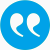#Böhm, Walter, Hornik, Kurt. 2010. A Kolmogorov-Smirnov Test for r Samples. Research Report Series, Institute for Statistics and Mathematics, Report 105.

BibTeX

## Abstract

We consider the problem of testing whether r (>=2) samples are drawn from the same continuous distribution F(x). The test statistic we will study in some detail is defined as the maximum of the circular differences of the empirical distribution functions, a generalization of the classical 2-sample Kolmogorov-Smirnov test to r (>=2) independent samples. For the case of equal sample sizes we derive the exact null distribution by counting lattice paths confined to stay in the scaled alcove $\mathcal{A}_r$ of the affine Weyl group $A_{r-1}$. This is done using a generalization of the classical reflection principle. By a standard diffusion scaling we derive also the asymptotic distribution of the test statistic in terms of a multivariate Dirichlet series. When the sample sizes are not equal the reflection principle no longer works, but we are able to establish a weak convergence result even in this case showing that by a proper rescaling a test statistic based on a linear transformation of the circular differences of the empirical distribution functions has the same asymptotic distribution as the test statistic in the case of equal sample sizes.(

## Tags

Press 'enter' for creating the tag

## Publication's profile

Status of publication Published WU Working/discussion paper, preprint English A Kolmogorov-Smirnov Test for r Samples Research Report Series, Institute for Statistics and Mathematics, Report 105 2010 http://epub.wu.ac.at/2960/

## Associations

People
Böhm, Walter (Details)
Hornik, Kurt (Details)
Organization
Institut f. Präsides SO (Details)
Institute for Statistics and Mathematics IN (Details)
Research Institute for Computational Methods FI (Details)